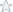Calculus Homework Assignment on Proofs

The questions for this homework assignment were as follows:

1. Determine whether the following statements are True or False. If False, provide a description (or theorem or picture or counterexample…) that explains your position.
A) If y=f(x) is increasing and differentiable and delta x>0, then delta y>dy.
B) The sum of two increasing functions is increasing.
C) If f1 is concave up and f2 is concave down on an interval I, then f1f2 is neither concave up nor concave down on I.

2. Let t(x)=tanx and notice that t(0)=t(pi)=0. Does there exist a number w for which t’(w)=0? Why or why not?
3. Consider the function h(t)=t-2sint on the interval [0,2pi]. Find all critical numbers of h, state the intervals of increase/decrease, and find all relative extrema.
4. Consider the function f(x)=x/x^2+1. State the intervals where the graph is concave upward/downward and find all points of inflection, if applicable.
5. The side of a cube is found to be 10cm long. From this, you find the volume of the cube is 10^3=1000cm^3. If your original measurement of the side is accurate to within 2%, approximately how accurate is your calculation of volume?
6. A box with a square base is constructed so the length of one side of the base plus the height is 10 inches. What is the largest possible volume of such a box?
7. Give a full analysis of the function y=x^3+3x^2+1. Include intercepts (approximate if necessary), asymptotes, intervals of increase/decrease, extrema, intervals of concavity, points of inflection, and a sketch. Note: some of this info may not apply to this function.

The solutions for part B and C for the first question are given below. Please purchase the full solutions if you wish to see the rest of the answers.

B
The sum of two increasing functions is increasing.

True

If f’(x) > 0 and g’(x) > 0
Then
f’(x) + g’(x) must be >0

C
If f1 is concave up and f2 is concave down on an interval I, then f1f2 is neither concave up nor concave down on I.

False

Take for example the following functions over the interval (-8,+8)
f(x) = x2 (which is concave/opens upward)
g(x) = -x2 (which is concave/opens downwards)
Observe that f(x)g(x) = -x2 is concave downwards over the same interval

Other Details about the Project/Assignment
Subjects: Mathematics -> Calculus
Topic: Homework Assignment on Calculus Proofs
Level: College / University
Tags:

Homework Assignment, Calculus Proofs

Price\$4.95

Math Genius
 Frederick Burke Member Since: March 2003 Customer Rating: 94.7% Projects Completed: 795 Total Earnings: *Private* +1 Ratings from clients: 115
Project Details
Subjects: Mathematics -> Calculus
Topic: Homework Assignment on Calculus Proofs
Level: College / University
Tags:

Homework Assignment, Calculus Proofs

Customer Reviews
Rated 2 Times
Rating( 4.5 / 5 Stars)

Reviews of the solutions left by other users
No customer has left a detailed review for these math solutions. Be the first one to purchase them and leave a review for others!

Not exactly what you are looking for?
We regularly update our math homework solutions library and are continually in the process of adding more samples and complete homework solution sets. If you do not find what you are looking for, just go ahead and place an order for a custom created homework solution. You can hire/pay a math genius to do your homework for you exactly to your specifications.